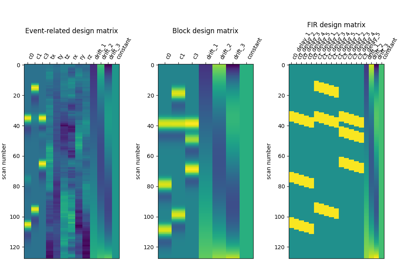Note

This page is a reference documentation. It only explains the function signature, and not how to use it. Please refer to the user guide for the big picture.

# 8.12.15.8. nilearn.glm.first_level.make_first_level_design_matrix¶

nilearn.glm.first_level.make_first_level_design_matrix(frame_times, events=None, hrf_model='glover', drift_model='cosine', high_pass=0.01, drift_order=1, fir_delays=, add_regs=None, add_reg_names=None, min_onset=- 24, oversampling=50)[source]

Generate a design matrix from the input parameters

Parameters
frame_timesarray of shape (n_frames,)

The timing of acquisition of the scans in seconds.

eventsDataFrame instance, optional
Events data that describes the experimental paradigm.
The DataFrame instance might have these keys:
‘onset’: column to specify the start time of each events in

seconds. An error is raised if this key is missing.

‘trial_type’: column to specify per-event experimental conditions

identifier. If missing each event are labelled ‘dummy’ and considered to form a unique condition.

‘duration’: column to specify the duration of each events in

seconds. If missing the duration of each events is set to zero.

‘modulation’: column to specify the amplitude of each

events. If missing the default is set to ones(n_events).

An experimental paradigm is valid if it has an ‘onset’ key and a ‘duration’ key. If these keys are missing an error will be raised. For the others keys a warning will be displayed. Particular attention should be given to the ‘trial_type’ key which defines the different conditions in the experimental paradigm.

hrf_model{‘glover’, ‘spm’, ‘spm + derivative’, ‘spm + derivative + dispersion’, ‘glover + derivative’, ‘glover + derivative + dispersion’, ‘fir’, None}, optional

Specifies the hemodynamic response function. Default=’glover’.

drift_model{‘cosine’, ‘polynomial’, None}, optional

Specifies the desired drift model. Default=’cosine’.

high_passfloat, optional

High-pass frequency in case of a cosine model (in Hz). Default=0.01.

drift_orderint, optional

Order of the drift model (in case it is polynomial). Default=1.

fir_delaysarray of shape(n_onsets) or list, optional

In case of FIR design, yields the array of delays used in the FIR model (in scans). Default=.

additional user-supplied regressors, e.g. data driven noise regressors or seed based regressors.

If None, while add_regs was provided, these will be termed ‘reg_%i’, i = 0..n_add_reg - 1 If add_regs is a DataFrame, the corresponding column names are used and add_reg_names is ignored.

min_onsetfloat, optional

Minimal onset relative to frame_times (in seconds) events that start before frame_times + min_onset are not considered. Default=-24.

oversamplingint, optional

Oversampling factor used in temporal convolutions. Default=50.

Returns
design_matrixDataFrame instance,

holding the computed design matrix, the index being the frames_times and each column a regressor.

## 8.12.15.8.1. Examples using `nilearn.glm.first_level.make_first_level_design_matrix`¶